GFG App
Open AppBrowser
Continue

# Uniform and Non-Uniform Motion along a Straight Line

The term “motion of a body” refers to the shift in a body’s position over a period of time. Some examples of objects exhibiting motion are a moving bus, the hands of a clock, the needle of a sewing machine, a cyclist cycling in a park, etc. Motion is mathematically defined in terms of distance, displacement, velocity, acceleration, and time. There are four types of motions, based on the nature of the movement of an object, namely linear motion, rotary motion, oscillatory motion, and reciprocating motion.

• Linear motion: The motion of a body along a straight line is known as the linear motion of a body. A man walking on a straight road, a swimmer swimming in a straight line, and the motion of a motorcycle on a straight road are some examples of linear motion.
• Rotary motion: Rotary motion is referred to as the rotation of a body around a fixed axis. The wheels of a moving bus, the blades of a fan, and the motion of a ferry wheel are some examples of rotary motion.
• Oscillatory motion: Oscillatory motion is referred to as the to-and-fro motion of a body about its mean position. A tuning fork, the motion of a swing, and a pendulum clock are some examples of oscillatory motion.
• Reciprocating motion: The reciprocating motion is a repetitive and continuous up and down or back and forth motion of a body. The needle of a sewing machine, an electric doorbell, and a shaping machine are some examples of reciprocating motion.

### Motion of a body along a straight line

The motion of a body along a straight line is known as the linear motion of a body. A man walking on a straight road, a swimmer swimming in a straight line, and the motion of a motorcycle on a straight road are some examples of linear motion. The linear motion of an object is further classified into two types based on the path of the object, i.e., rectilinear motion or curvilinear motion.

• Rectilinear motion: When an object that is moving in a linear motion opts for a straight-line path, then the object is said to be in rectilinear motion. The motion of a train on a straight track is an example of rectilinear motion.
• Curvilinear motion: When an object that is moving in a linear motion opts for a curved path, then the object is said to be in a curvilinear motion. The motion of a ball thrown into the air at an angle is an example of curvilinear motion.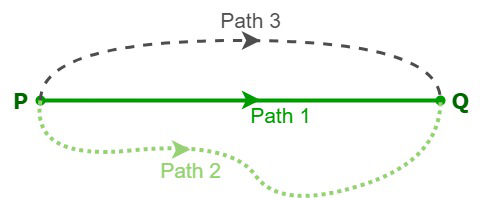For example, an object traveled from point P to point Q in three paths. Path 1 is a rectilinear motion, whereas paths 2 and 3 are curvilinear motions.

### Uniform and non-uniform motion of a body along a straight line

• Uniform motion: The uniform motion of a body can be defined as the motion of a body that travels with constant velocity along a straight line and also covers equal distances in equal intervals of time, irrespective of the time duration. As the body is moving in rectilinear motion with a constant velocity, the acceleration of the body is zero.

Examples: Let us suppose that a car is traveling at a speed of 15 m/s, which means the car covers 15 meters in one second. Hence, the speed of the car is constant for every second and its acceleration is zero. The movement of the blades of a ceiling fan, the blades of a turbine, and windmills are other examples of uniform motion.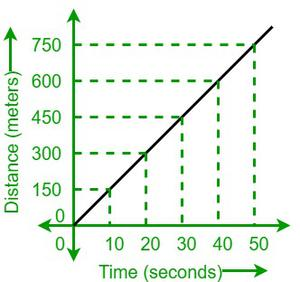Uniform motion distance-time graph.

• Non-uniform motion: The non-uniform motion of a body is defined as the motion of a body that travels at odd speeds and doesn’t cover equal distances at equal time intervals, irrespective of the duration of time. As the body is moving in rectilinear motion and the motion of the body is not consistent, the acceleration of the body will be non-zero.

Examples: A cyclist traveled 50 meters in the first 5 seconds and 100 meters in the next 5 seconds. This shows the inconsistent speed of a cyclist. The motion of a train is another example of non-uniform motion.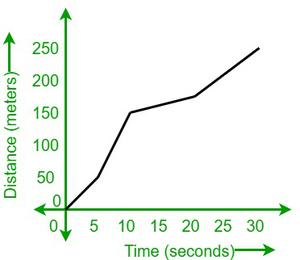Non-uniform motion distance-time graph.

### Sample Questions

Question 1: Define the motion of a body and linear motion with examples.

The term “motion of a body” refers to the shift in a body’s position over a period of time. The motion of a body along a straight line is known as the linear motion of a body. A man walking on a straight road, a swimmer swimming in a straight line, and the motion of a motorcycle on a straight road are some examples of linear motion.

Question 2: Write the differences between the uniform and non-uniform motion of a body along a straight line.

Question 3: Define uniform motion with an example.

The uniform motion of a body can be defined as the motion of a body that travels with constant velocity along a straight line and also covers equal distances in equal intervals of time, irrespective of the time duration. As the body is moving in rectilinear motion with a constant velocity, the acceleration of the body is zero.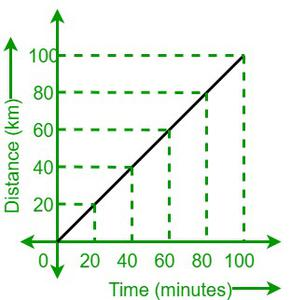Example: Let’s suppose that a bus is traveling at a speed of 60km/h, which means the bus covers 60 km in one hour, i.e., 1 km in one minute. Hence, the speed of the bus is constant for every second and its acceleration is zero. The movement of the blades of a ceiling fan, the blades of a turbine, and windmills are some examples of uniform motion.

Question 4: What is linear motion and what are the different types of linear motion?

The motion of a body along a straight line is known as the linear motion of a body. The linear motion of an object is further classified into two types based on the path of the object, i.e., rectilinear motion or curvilinear motion.

Rectilinear motion: When an object that is moving in a linear motion opts for a straight-line path, then the object is said to be in rectilinear motion. The motion of a train on a straight track is an example of rectilinear motion.

Curvilinear motion: When an object that is moving in a linear motion opts for a curved path, then the object is said to be in a curvilinear motion. The motion of a ball thrown into the air at an angle is an example of curvilinear motion.

Question 5: Define non-uniform motion with an example.

The non-uniform motion of a body is defined as the motion of a body that travels at odd speeds and doesn’t cover equal distances at equal time intervals, irrespective of the duration of time. As the body is moving in rectilinear motion and the motion of the body is not consistent, the acceleration of the body will be non-zero.

Example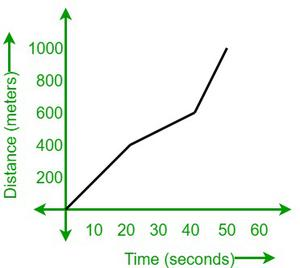A car travels 400 meters in 20 seconds, i.e., its speed is 20 m/s. In the next 20 seconds, it covered 200 meters, i.e., the speed of the car was 10 m/s. The speed of the car in two equal time intervals is different. This shows the inconsistent speed of the car.

My Personal Notes arrow_drop_up